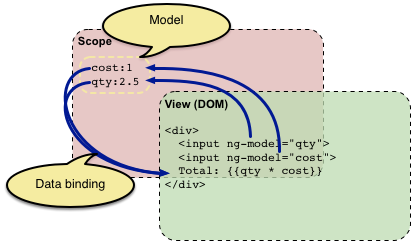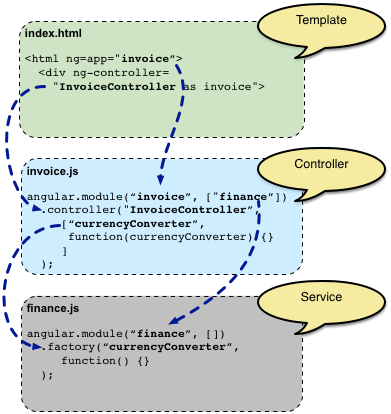Geeks With Blogs
Conceptual Overview
This section briefly touches on all of the important parts of AngularJS using a simple example.
 Concept Description Template HTML with additional markup Directives extend HTML with custom attributes and elements Model the data shown to the user in the view and with which the user interacts Scope context where the model is stored so that controllers, directives and expressions can access it Expressions access variables and functions from the scope Compiler parses the template and instantiates directives and expressions Filter formats the value of an expression for display to the user View what the user sees (the DOM) Data Binding sync data between the model and the view Controller the business logic behind views Dependency Injection Creates and wires objects and functions Injector dependency injection container Module a container for the different parts of an app including controllers, services, filters, directives which configures the Injector Service reusable business logic independent of views
A first example: Data binding
In the following example we will build a form to calculate the costs of an invoice in different currencies.
Let's start with input fields for quantity and cost whose values are multiplied to produce the total of the invoice:
Our index page will be like this :
`````` <div ng-app ng-init="qty=1;cost=2">
<b>Invoice:</b>
<div>
Quantity: <input type="number" ng-model="qty" required >
</div>
<div>
Costs: <input type="number" ng-model="cost" required >
</div>
<div>
<b>Total:</b> {{qty * cost | currency}}
</div>
</div>
``````
`````` Invoice:
Quantity: 1
Costs: 2
Total: \$2.00
``````
Let's walk through the example and describe what's going on.
This looks like normal HTML, with some new markup. In Angular, a file like this is called a "template". When Angular starts your application, it parses and processes this new markup from the template using the so called "compiler". The loaded, transformed and rendered DOM is then called the "view".The first kind of new markup are the so called "directives". They apply special behavior to attributes or elements in the HTML. In the example above we use the ng-app attribute, which is linked to a directive that automatically initializes our application. Angular also defines a directive for the input element that adds extra behavior to the element. E.g. it is able to automatically validate that the entered text is non empty by evaluating the required attribute. The ng-model directive stores/updates the value of the input field into/from a variable and shows the validation state of the input field by adding css classes. In the example we use these css classes to mark an empty input field with a red border.
The second kind of new markup are the double curly braces {{ expression | filter }}: When the compiler encounters this markup, it will replace it with the evaluated value of the markup. An "expression" in a template is a JavaScript-like code snippet that allows to read and write variables. Note that those variables are not global variables. Just like variables in a JavaScript function live in a scope, Angular provides a "scope" for the variables accessible to expressions. The values that are stored in variables on the scope are referred to as the "model" in the rest of the documentation. Applied to the example above, the markup directs Angular to "take the data we got from the input widgets and multiply them together".
The example above also contains a "filter". A filter formats the value of an expression for display to the user. In the example above, the filter currency formats a number into an output that looks like money.
The important thing in the example is that angular provides live bindings: Whenever the input values change, the value of the expressions are automatically recalculated and the DOM is updated with their values. The concept behind this is "two-way data binding".
Now our index page code will be :
`````` <div ng-app="invoice" ng-controller="InvoiceController as invoice">
<b>Invoice:</b>
<div>
Quantity: <input type="number" ng-model="invoice.qty" required >
</div>
<div>
Costs: <input type="number" ng-model="invoice.cost" required >
<select ng-model="invoice.inCurr">
<option ng-repeat="c in invoice.currencies">{{c}}</option>
</select>
</div>
<div>
<b>Total:</b>
<span ng-repeat="c in invoice.currencies">
{{invoice.total(c) | currency:c}}
</span>
<button class="btn" ng-click="invoice.pay()">Pay</button>
</div>
</div>
``````
``//invoice.js code snippet :``
`````` angular.module('invoice', [])
.controller('InvoiceController', function() {
this.qty = 1;
this.cost = 2;
this.inCurr = 'EUR';
this.currencies = ['USD', 'EUR', 'CNY'];
this.usdToForeignRates = {
USD: 1,
EUR: 0.74,
CNY: 6.09
};
this.total = function total(outCurr) {
return this.convertCurrency(this.qty * this.cost, this.inCurr, outCurr);
};
this.convertCurrency = function convertCurrency(amount, inCurr, outCurr) {
return amount * this.usdToForeignRates[outCurr] / this.usdToForeignRates[inCurr];
};
this.pay = function pay() {
};
});
``````
What changed?
First, there is a new JavaScript file that contains a so called "controller". More exactly, the file contains a constructor function that creates the actual controller instance. The purpose of controllers is to expose variables and functionality to expressions and directives.
Besides the new file that contains the controller code we also added a ng-controller directive to the HTML. This directive tells angular that the new InvoiceController is responsible for the element with the directive and all of the element's children. The syntax InvoiceController as invoice tells Angular to instantiate the controller and save it in the variable invoice in the current scope.
We also changed all expressions in the page to read and write variables within that controller instance by prefixing them with invoice. . The possible currencies are defined in the controller and added to the template using ng-repeat. As the controller contains a total function we are also able to bind the result of that function to the DOM using {{ invoice.total(...) }}.
Again, this binding is live, i.e. the DOM will be automatically updated whenever the result of the function changes. The button to pay the invoice uses the directive ngClick. This will evaluate the corresponding expression whenever the button is clicked.
In the new JavaScript file we are also creating a module at which we register the controller. We will talk about modules in the next section.
The following graphic shows how everything works together after we introduced the controller:Right now, the InvoiceController contains all logic of our example. When the application grows it is a good practice to move view independent logic from the controller into a so called "service", so it can be reused by other parts of the application as well. Later on, we could also change that service to load the exchange rates from the web, e.g. by calling the Yahoo Finance API, without changing the controller.
Let's refactor our example and move the currency conversion into a service in another file:
invoice.js code snippet :
`````` angular.module('invoice', ['finance'])
.controller('InvoiceController', ['currencyConverter', function(currencyConverter) {
this.qty = 1;
this.cost = 2;
this.inCurr = 'EUR';
this.currencies = currencyConverter.currencies;
this.total = function total(outCurr) {
return currencyConverter.convert(this.qty * this.cost, this.inCurr, outCurr);
};
this.pay = function pay() {
};
}]);
``````
finace.js code snippet :
`````` angular.module('finance', [])
.factory('currencyConverter', function() {
var currencies = ['USD', 'EUR', 'CNY'];
var usdToForeignRates = {
USD: 1,
EUR: 0.74,
CNY: 6.09
};
var convert = function (amount, inCurr, outCurr) {
return amount * usdToForeignRates[outCurr] / usdToForeignRates[inCurr];
};
return {
currencies: currencies,
convert: convert
};
});
``````
What changed?
We moved the convertCurrency function and the definition of the existing currencies into the new file finance.js. But how does the controller get a hold of the now separated function?
This is where "Dependency Injection" comes into play. Dependency Injection (DI) is a software design pattern that deals with how objects and functions get created and how they get a hold of their dependencies. Everything within Angular (directives, filters, controllers, services, ...) is created and wired using dependency injection. Within Angular, the DI container is called the "injector".
To use DI, there needs to be a place where all the things that should work together are registered. In Angular, this is the purpose of the so called "modules". When Angular starts, it will use the configuration of the module with the name defined by the `ng-app` directive, including the configuration of all modules that this module depends on.In the example above: The template contains the directive ng-app="invoice". This tells Angular to use the invoice module as the main module for the application. The code snippet angular.module('invoice', ['finance']) specifies that the invoice module depends on the finance module. By this, Angular uses the InvoiceController as well as the currencyConverter service.
Now that Angular knows of all the parts of the application, it needs to create them. In the previous section we saw that controllers are created using a factory function. For services there are multiple ways to define their factory (see the service guide). In the example above, we are using a function that returns the currencyConverter function as the factory for the service.
Back to the initial question: How does the `InvoiceController` get a reference to the `currencyConverter` function? In Angular, this is done by simply defining arguments on the constructor function. With this, the injector is able to create the objects in the right order and pass the previously created objects into the factories of the objects that depend on them. In our example, the `InvoiceController` has an argument named `currencyConverter`. By this, Angular knows about the dependency between the controller and the service and calls the controller with the service instance as argument.
The last thing that changed in the example between the previous section and this section is that we now pass an array to the module.controller function, instead of a plain function. The array first contains the names of the service dependencies that the controller needs. The last entry in the array is the controller constructor function. Angular uses this array syntax to define the dependencies so that the DI also works after minifying the code, which will most probably rename the argument name of the controller constructor function to something shorter like a.
Accessing the backend
Let's finish our example by fetching the exchange rates from the Yahoo Finance API. The following example shows how this is done with Angular:
Now the invoice.js code snippet will be like this :
`````` angular.module('invoice', ['finance'])
.controller('InvoiceController', ['currencyConverter', function(currencyConverter) {
this.qty = 1;
this.cost = 2;
this.inCurr = 'EUR';
this.currencies = currencyConverter.currencies;
this.total = function total(outCurr) {
return currencyConverter.convert(this.qty * this.cost, this.inCurr, outCurr);
};
this.pay = function pay() {
};
}]);
``````
And the finance.js code snippet :
`````` angular.module('finance', [])
.factory('currencyConverter', ['\$http', function(\$http) {
var YAHOO_FINANCE_URL_PATTERN =
'http://query.yahooapis.com/v1/public/yql?q=select * from '+
'yahoo.finance.xchange where pair in ("PAIRS")&format=json&'+
'env=store://datatables.org/alltableswithkeys&callback=JSON_CALLBACK';
var currencies = ['USD', 'EUR', 'CNY'];
var usdToForeignRates = {};
var convert = function (amount, inCurr, outCurr) {
return amount * usdToForeignRates[outCurr] / usdToForeignRates[inCurr];
};
var refresh = function() {
var url = YAHOO_FINANCE_URL_PATTERN.
replace('PAIRS', 'USD' + currencies.join('","USD'));
return \$http.jsonp(url).success(function(data) {
var newUsdToForeignRates = {};
angular.forEach(data.query.results.rate, function(rate) {
var currency = rate.id.substring(3,6);
newUsdToForeignRates[currency] = window.parseFloat(rate.Rate);
});
usdToForeignRates = newUsdToForeignRates;
});
};
refresh();
return {
currencies: currencies,
convert: convert,
refresh: refresh
};
}]);
``````
What changed?
Our currencyConverter service of the finance module now uses the \$http, a built-in service provided by Angular for accessing a server backend. \$http is a wrapper around XMLHttpRequest and JSONP transports.